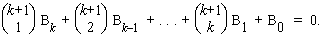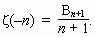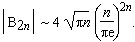# Bernoulli number

The Bernoulli numbers come from the coefficients in the Taylor expansion of x/(ex-1). They can be defined recursively by setting B0=1, and then usingThe first few Bernoulli numbers are B0=1, B1=-1/2, B2=1/6, B3=0, B4=-1/30, B5=0, B6=1/42, B7=0, B8=-1/30, B9=0, and B10=5/66. Notice that all of the odd terms, B2n+1 (n > 1), are zero; and the even terms alternate in sign.

These numbers can also be defined using the Riemann zeta function as followsFinally, using Stirling's formula, we haveThe Bernoulli numbers first appeared in the posthumous work "Ars Conjectandi" (1713) by Jakob Bernoulli. Euler used them to express the sums of equal powers of consecutive integers. They also are important in classical assaults of Fermat's Last Theorem.